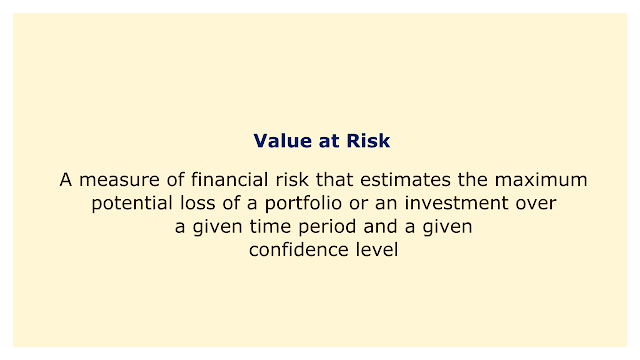# Value at RiskImage: Moneybestpal.com

### Value at risk (VaR) is a commonly used indicator of financial risk that calculates the largest possible loss that could occur with respect to a portfolio or investment over a certain time frame and degree of confidence.

VaR is helpful for determining risk caps and capital needs, as well as for evaluating the exposure and performance of various assets, strategies, and portfolios.

Numerous techniques, including historical simulation, parametric methods, and Monte Carlo simulation, can be used to calculate VaR. Depending on the assumptions and the availability of data, each method has pros and cons of its own. The nature and complexity of the portfolio, the risk factors involved, and the required level of accuracy and robustness all affect the technique selection.

The main steps to calculate VaR are:
• Specify the investment or portfolio to be examined.
• Set the time frame and level of assurance for the VaR estimation.
• Determine and measure the pertinent risk elements affecting the investment or portfolio.
• Select a technique to determine how the returns on a portfolio or investment are distributed.
• Determine the VaR by taking the quantile of the distribution that corresponds to the level of confidence.

Consider the scenario where we want to calculate the VaR of a stock portfolio over a one-day period with a 95% confidence level. To calculate the distribution of the portfolio returns based on historical data, we can utilize historical simulation. The 5th percentile value can then be determined by ordering the returns from lowest to highest. The portfolio's VaR is represented by this number, which indicates that there is a 5% possibility that it will lose more than this much in a single day.
Tags